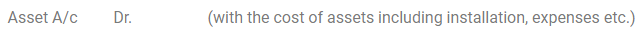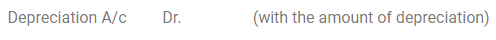# Methods of Recording Depreciation

Methods of Recording Depreciation

For recording depreciation on fixed assets there are two types of arrangements:

• Charging depreciation to the asset account or,
• Creating Provision for the depreciation/Accumulated depreciation account.

(A) Charging depreciation to asset account: As per this arrangement, depreciation is directly deducted from the depreciable cost of the asset, i.e., book value of the asset declines (credited to the asset account) and charged (or debited) to the profit and loss account. Journal entries under this recording technique are as follows:

1. Upon purchase of asset by the business concern purchase of assetTo Bank/Vendor A/c

2. Following two entries are recorded at the end of every year

(a) For charging depreciation amount to the asset account

Depreciation A/c Dr. (with the amount of depreciation)

To Asset A/c

(b) For booking depreciation expense to the profit and loss account.

Profit and Loss A/c     Dr. (with the amount of depreciation)

To Depreciation A/c

3. Balance Sheet Treatment

When this technique is utilized, the fixed asset shows up at its net book value (i.e. depreciation is deducted from the asset balance) on the asset side of the balance sheet and not at its original cost (otherwise called historical cost).

(B) Creating Provision for Depreciation Account/Accumulated Depreciation Account:

This technique is intended to accumulate the depreciation provided on an asset in a different account generally called Provision for Depreciation or Accumulated Depreciation account.

By such accumulation of depreciation the asset account need not be changed at all and it keeps on being appeared at its original cost over the progressive years of its valuable life. There are some fundamental characteristics of this technique for recording depreciation. These are given underneath:

• Asset account continuously appears at its original cost year after year over its entire valuable life;
• Depreciation is accumulated on a separate account instead of being adjusted directly in the asset account as we did in the previous method.

The following journal entries are recorded under this technique:

1. Upon purchase of asset by the business concern purchase of assetTo Bank/Vendor A/c (cash/credit purchase)

2. To record depreciation, these journal entries are recorded at the end of each year:

(a) For recording depreciation amount in the provision for depreciation accountTo Provision for depreciation A/c

(b) For booking depreciation expense to the profit and loss account.To Depreciation A/c

3. Balance sheet treatment

In the balance sheet, the fixed asset keeps on showing up at its original cost on the asset side. The depreciation charged till that date shows up in the provision for depreciation account, which is demonstrated either on the liabilities side of the balance sheet or by method of deduction from the original cost of the asset concerned on the asset side of the balance sheet.

Thus, an organisation can choose to record the depreciation by following any of the two methods of recording depreciation. It can either charge depreciation to the asset account itself or can create a separate provision for depreciation account.